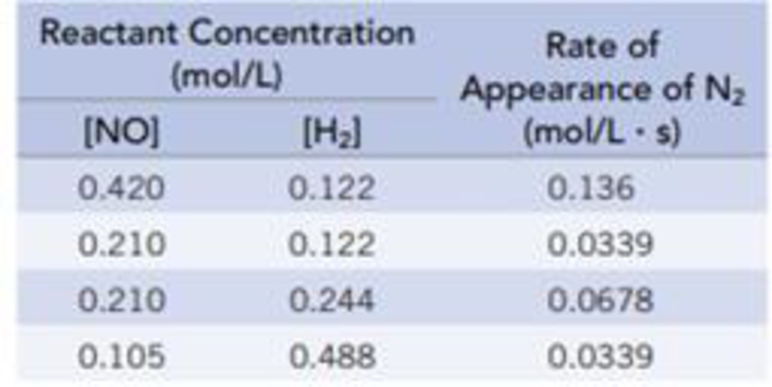# The reaction 2 NO(g) + 2 H 2 (g) → N 2 (g) + 2 H 2 O(g) was studied at 904 °C, and the data in the table were collected. (a) Determine the order of the reaction for each reactant. (b) Write the rate equation for the reaction. (c) Calculate the rate constant for the reaction. (d) Find the rate of appearance of N 2 at the instant when [NO] = 0.350 mol/L and [H] = 0.205 mol/L.### Chemistry & Chemical Reactivity

9th Edition
John C. Kotz + 3 others
Publisher: Cengage Learning
ISBN: 9781133949640

#### Solutions

Chapter
Section### Chemistry & Chemical Reactivity

9th Edition
John C. Kotz + 3 others
Publisher: Cengage Learning
ISBN: 9781133949640
Chapter 14, Problem 12PS
Textbook Problem
52 views

## The reaction2 NO(g) + 2 H2(g) → N2(g) + 2 H2O(g)was studied at 904 °C, and the data in the table were collected.(a) Determine the order of the reaction for each reactant. (b) Write the rate equation for the reaction. (c) Calculate the rate constant for the reaction. (d) Find the rate of appearance of N2 at the instant when [NO] = 0.350 mol/L and [H] = 0.205 mol/L.

(a)

Interpretation Introduction

Interpretation: The order of the reaction for each reactant has to be calculated.

Concept introduction:

Rate law or rate equation: The relationship between the reactant concentrations and reaction rate is expressed by an equation.

aA + bBxXRate of reaction = k [A]m[B]n

Order of a reaction: The order of a reaction with respect to a particular reactant is the exponent of its concentration term in the rate law expression, and the overall reaction order is the sum of the exponents on all concentration terms.

Rate constant, k: It is a proportionality constant that relates rate and concentration at a given temperature.

### Explanation of Solution

The order of the reaction is calculated as,

Reaction Rate = k [NO]m[H2]n,where m, and n are orders of the reactants.Givenreaction: 2 NO(g) + 2 H2(g)®N2(g)+ 2 H2(g)Findorderofthereaction:_Comparingfirsttwoexperiments1and2,rate1=[NO]m[H2]n, rate1 = 0.136 mol/L.srate2 = k [NO]m[H2]n, rate2 = 0.0339 mol/L.srate1rate2=k [NO]m[H2]nk [NO]m[H2]n0.136mol/L.s0.0339mol/L.s=(0.420)m(0.122)n(0.210)m(0

(b)

Interpretation Introduction

Interpretation: The rate of the reaction has to be written.

Concept introduction:

Rate law or rate equation: The relationship between the reactant concentrations and reaction rate is expressed by an equation.

aA + bBxXRate of reaction = k [A]m[B]n

Order of a reaction: The order of a reaction with respect to a particular reactant is the exponent of its concentration term in the rate law expression, and the overall reaction order is the sum of the exponents on all concentration terms.

Rate constant, k: It is a proportionality constant that relates rate and concentration at a given temperature.

(c)

Interpretation Introduction

Interpretation: The order of the reaction for each reactant has to be calculated.

Concept introduction:

Rate law or rate equation: The relationship between the reactant concentrations and reaction rate is expressed by an equation.

aA + bBxXRate of reaction = k [A]m[B]n

Order of a reaction: The order of a reaction with respect to a particular reactant is the exponent of its concentration term in the rate law expression, and the overall reaction order is the sum of the exponents on all concentration terms.

Rate constant, k: It is a proportionality constant that relates rate and concentration at a given temperature.

(d)

Interpretation Introduction

Interpretation:

The rate of appearance of N2 at the instant has to be found.

Concept introduction:

Rate law or rate equation: The relationship between the reactant concentrations and reaction rate is expressed by an equation.

aA + bBxXRate of reaction = k [A]m[B]n

Order of a reaction: The order of a reaction with respect to a particular reactant is the exponent of its concentration term in the rate law expression, and the overall reaction order is the sum of the exponents on all concentration terms.

Rate constant, k: It is a proportionality constant that relates rate and concentration at a given temperature.

### Still sussing out bartleby?

Check out a sample textbook solution.

See a sample solution

#### The Solution to Your Study Problems

Bartleby provides explanations to thousands of textbook problems written by our experts, many with advanced degrees!

Get Started

Find more solutions based on key concepts
The nervous system sends messages to the glands, telling them what to do. T F

Nutrition: Concepts and Controversies - Standalone book (MindTap Course List)

Explain the acronyms mks, SI, and cgs.

An Introduction to Physical Science

What gives amino acids their individual properties?

Biology: The Dynamic Science (MindTap Course List)

What is genomics?

Human Heredity: Principles and Issues (MindTap Course List)

True or false? Ferns produce seeds inside sori.

Biology: The Unity and Diversity of Life (MindTap Course List)

A light ray containing both blue and red wavelengths is incident at an angle on a slab of glass. Which of the s...

Physics for Scientists and Engineers, Technology Update (No access codes included)

Whats a monsoon? Do we experience monsoons in the continental United States?

Oceanography: An Invitation To Marine Science, Loose-leaf Versin